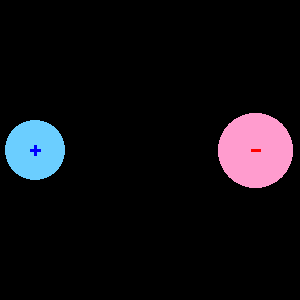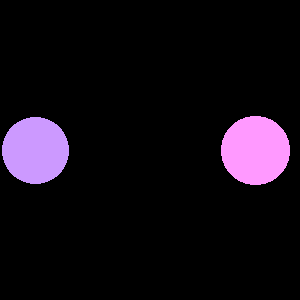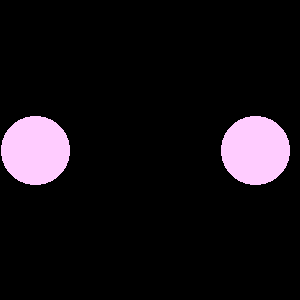# Dipole Moment

Let's go back to that first ion pair which was formed when the positive ion and the negative ion came together. If the electronegativities of the elements are sufficiently different (like an alkali metal and a halide), the charges on the paired ions will not change appreciably - there will be a full electron charge on the blue ion and a full positive charge on the red ion. The bond formed by the attraction of these opposite charges is called an ionic bond. If the difference in electronegativity is not so great, however, there will be some degree of sharing of the electrons between the two atoms. The result is the same whether two ions come together or two atoms come together:Polar Molecule

The combination of atoms or ions is no longer a pair of ions, but rather a polar molecule which has a measureable dipole moment. The dipole moment (D) is defined as if there were a positive (+q) and a negative (-q) charge separated by a distance (r):

$D = qr$

If there is no difference in electronegativity between the atoms (as in a diatomic molecule such as $$O_2$$ or $$F_2$$) there is no difference in charge and no dipole moment. The bond is called a covalent bond, the molecule has no dipole moment, and the molecule is said to be non-polar. Bonds between different atoms have different degrees of ionicity depending on the difference in the electronegativities of the atoms. The degree of ionicity may range from zero (for a covalent bond between two atoms with the same electronegativity) to one (for an ionic bond in which one atom has the full charge of an electron and the other atom has the opposite charge).Figure 2: Non-polar Molecule

In some cases, two or more partially ionic bonds arranged symmetrically around a central atom may mutually cancel each other's polarity, resulting in a non-polar molecule. An example of this is seen in the carbon tetrachloride ($$CCl_4$$) molecule. There is a substantial difference between the electronegativities of carbon (2.55) and chlorine (3.16), but the four chlorine atoms are arranged symmetrically about the carbon atom in a tetrahedral configuration, and the molecule has zero dipole moment. Saturated hydrocarbons ($$C_nH_{n+2}$$) are non-polar molecules because of the small difference in the electronegativities of carbon and hydrogen plus the near symmetry about each carbon atom.